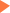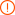# SAT数学考试中的常用逻辑符号：A ⇔ B 是啥意思呢?

2018年03月14日15:28 来源：小站整理## SAT/ACT备考资料免费领取 请选择资料！

SAT数学考试中，或多或少的都会经常出现一些常用逻辑符号，感觉有点眼生，怎么办？那我们就来看看这些逻辑符号到底是什么意思吧~

A ⇒ B means if A is true then B is also true; if A is false then nothing is said about B.

→ may mean the same as ⇒ (the symbol may also indicate the domain and codomain of a function; see table of mathematical symbols).

⊃ may mean the same as ⇒ (the symbol may also mean superset).

A ⇔ B means A is true if B is true and A is false if B is false.

The statement ¬A is true if and only if A is false.

The statement A ∧ B is true if A and B are both true; else it is false.

The statement A ∨ B is true if A or B (or both) are true; if both are false, the statement is false.

∀ x: P(x) means P(x) is true for all x.

∃ x: P(x) means there is at least one x such that P(x) is true.

∃! x: P(x) means there is exactly one x such that P(x) is true.

x ⊢ y means y is derived from x.

### 专题推荐未输入验证码未输入验证码未输入验证码

## SAT30天热搜沪公网安备 31010602002658号